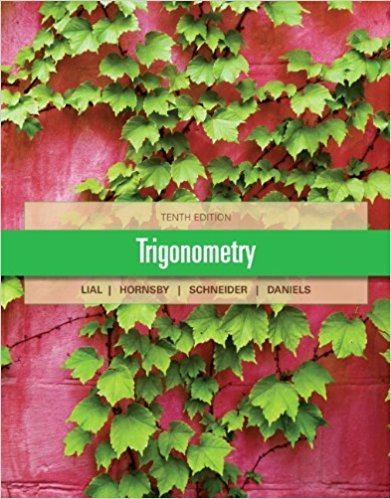×
×

# Solutions for Chapter 4.4: Graphs of the Secant and Cosecant Functions## Full solutions for Trigonometry | 10th Edition

ISBN: 9780321671776Solutions for Chapter 4.4: Graphs of the Secant and Cosecant Functions

Solutions for Chapter 4.4
4 5 0 326 Reviews
25
5
##### ISBN: 9780321671776

Trigonometry was written by and is associated to the ISBN: 9780321671776. This expansive textbook survival guide covers the following chapters and their solutions. Since 36 problems in chapter 4.4: Graphs of the Secant and Cosecant Functions have been answered, more than 34275 students have viewed full step-by-step solutions from this chapter. Chapter 4.4: Graphs of the Secant and Cosecant Functions includes 36 full step-by-step solutions. This textbook survival guide was created for the textbook: Trigonometry, edition: 10.

Key Math Terms and definitions covered in this textbook
• Affine transformation

Tv = Av + Vo = linear transformation plus shift.

• Cayley-Hamilton Theorem.

peA) = det(A - AI) has peA) = zero matrix.

• Column picture of Ax = b.

The vector b becomes a combination of the columns of A. The system is solvable only when b is in the column space C (A).

• Complete solution x = x p + Xn to Ax = b.

(Particular x p) + (x n in nullspace).

• Distributive Law

A(B + C) = AB + AC. Add then multiply, or mUltiply then add.

• Hermitian matrix A H = AT = A.

Complex analog a j i = aU of a symmetric matrix.

• Left inverse A+.

If A has full column rank n, then A+ = (AT A)-I AT has A+ A = In.

• Linear transformation T.

Each vector V in the input space transforms to T (v) in the output space, and linearity requires T(cv + dw) = c T(v) + d T(w). Examples: Matrix multiplication A v, differentiation and integration in function space.

• Linearly dependent VI, ... , Vn.

A combination other than all Ci = 0 gives L Ci Vi = O.

• Multiplicities AM and G M.

The algebraic multiplicity A M of A is the number of times A appears as a root of det(A - AI) = O. The geometric multiplicity GM is the number of independent eigenvectors for A (= dimension of the eigenspace).

• Orthogonal matrix Q.

Square matrix with orthonormal columns, so QT = Q-l. Preserves length and angles, IIQxll = IIxll and (QX)T(Qy) = xTy. AlllAI = 1, with orthogonal eigenvectors. Examples: Rotation, reflection, permutation.

• Orthonormal vectors q 1 , ... , q n·

Dot products are q T q j = 0 if i =1= j and q T q i = 1. The matrix Q with these orthonormal columns has Q T Q = I. If m = n then Q T = Q -1 and q 1 ' ... , q n is an orthonormal basis for Rn : every v = L (v T q j )q j •

• Projection p = a(aTblaTa) onto the line through a.

P = aaT laTa has rank l.

• Random matrix rand(n) or randn(n).

MATLAB creates a matrix with random entries, uniformly distributed on [0 1] for rand and standard normal distribution for randn.

• Rank r (A)

= number of pivots = dimension of column space = dimension of row space.

• Schwarz inequality

Iv·wl < IIvll IIwll.Then IvTAwl2 < (vT Av)(wT Aw) for pos def A.

• Simplex method for linear programming.

The minimum cost vector x * is found by moving from comer to lower cost comer along the edges of the feasible set (where the constraints Ax = b and x > 0 are satisfied). Minimum cost at a comer!

• Solvable system Ax = b.

The right side b is in the column space of A.

• Standard basis for Rn.

Columns of n by n identity matrix (written i ,j ,k in R3).